## ML Aggarwal Class 8 Solutions for ICSE Maths Chapter 19 Data Handling Ex 19.2

Question 1.
The following data represents the different number of animals in a zoo. Prepare a pie chart for the given data.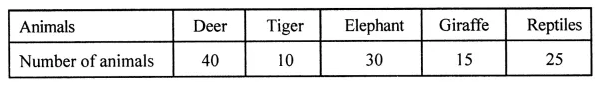Solution: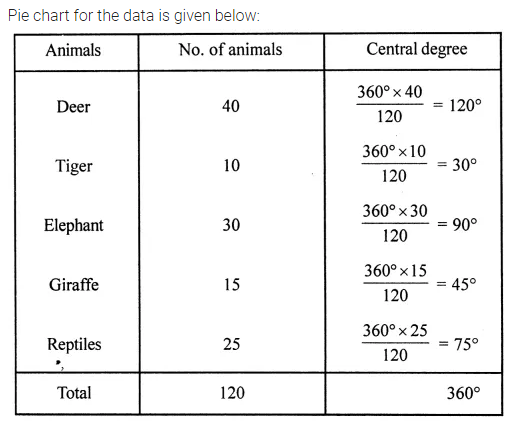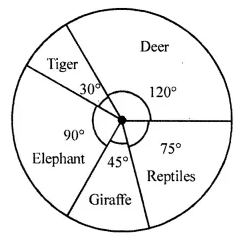Question 2.
The following data represents the monthly expenditure of a family (in T) on various items. Draw a pie chart to represent this data.Solution: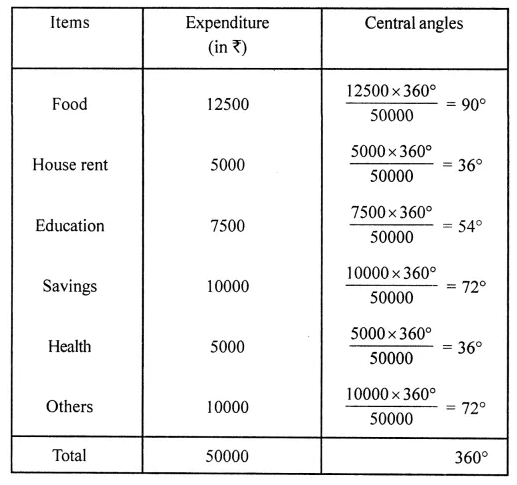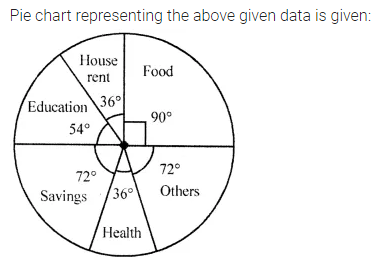Question 3.
The following data represents the percentage distribution of the expenditure incurred in publishing a book.Draw a pie chart to represent this data.
Solution: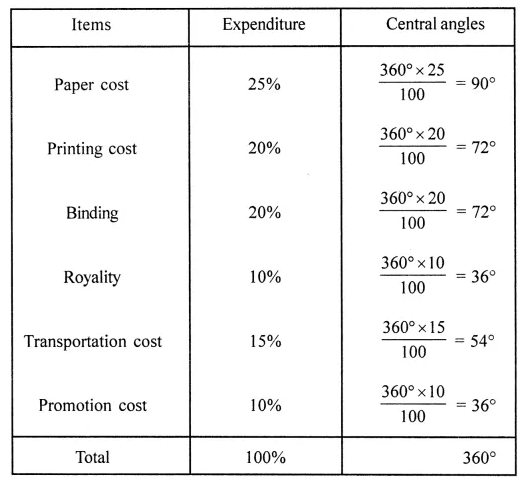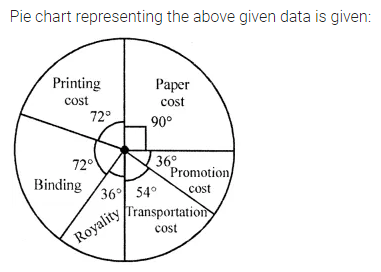Question 4.
The following data represents the number of students got admission in different streams of a college: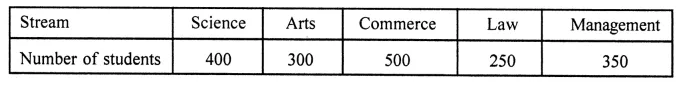Draw a pie chart to represent this data.
Solution: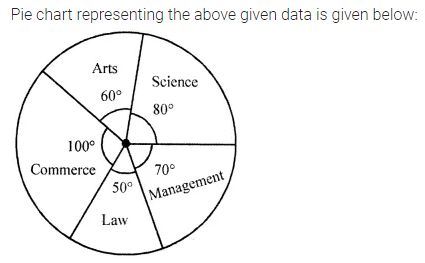Question 5.
The adjoining pie chart shows the expenditure of a country on various sports during year 2012. Study the pie chart carefully and answer the following questions: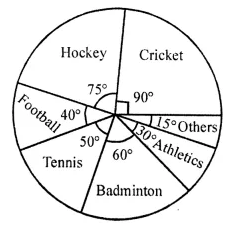(i) What percent of total expenditure is spent on cricket?
(ii) How much percent more is spent on hockey than that on tennis?
(iii) If the total amount spent on sports in 2012 is ₹1,80,00,000, then find amount spent on Badminton,
(iv) If the total amount spent on sports in 2012 is ₹2,40,00,000 then find the amount spent on cricket and hockey together.
Solution: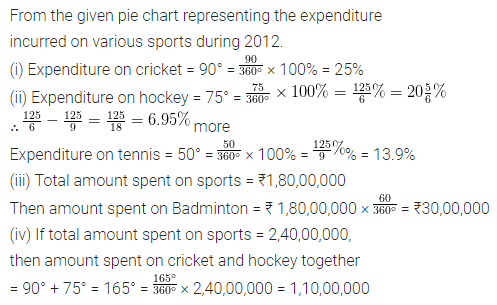Question 6.
The adjoining pie chart shows the number of students enrolled in class VI to class X of a school.If 1440 students are enrolled from VI to X, then answer the following questions:
(i) How many students are enrolled in class VIII?
(ii) How many students are more in class IX than in class X?
(iii) What is the sum of students enrolled in VII and VIII?
(iv) Find the ratio of students enrolled in VI to students enrolled in X.
Solution: